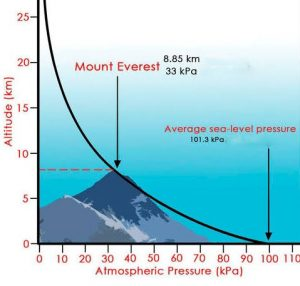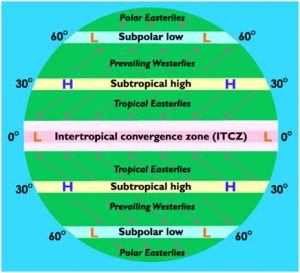Latest SSC jobs   »   GK Questions   »   Atmospheric Pressure

# What is Atmospheric Pressure? Formula and Value

## What is Atmospheric Pressure?

The weight of a column of air contained in a unit area from the mean sea level to the top of the atmosphere is called the atmospheric pressure(definition of atmospheric pressure).

• It is measured in force per unit area.
• Atmospheric Pressure is expressed in ‘milibar’ or mb. For application purpose, it is also measured in Kilo Pascal.
• Instruments used to measure Atmospheric Pressure :
The aneroid barometer or mercury barometer is the instrument used to measure Atmospheric pressure.
• The rate of change of pressure in regard to distance is the pressure gradient.

## Vertical Variation of Atmospheric pressure

In the lower atmosphere the pressure decreases rapidly with height. The decrease in pressure with altitude, however, is not constant. Since the factors controlling air density – temperature, amount of water vapor and gravity are variable, there is no simple relationship between altitude and pressure.In general, the atmospheric pressure decreases on an average at the rate of about 34 millibars every 300 metres of height. The vertical pressure gradient force is much larger than that of the horizontal pressure gradient. But, it is generally balanced by a nearly equal but opposite gravitational force. Hence, we do not experience strong upward winds.
The low-pressure system is encircled by one or more isobars with the lowest pressure at the centre.

## Horizontal Distribution of Atmospheric Pressure

• Horizontal distribution of pressure is studied by drawing isobars at constant levels.
• Isobars are lines connecting places having equal pressure. In order to eliminate the effect of altitude on pressure, it is measured at any station after being reduced to sea level for purposes of comparison.
• There are distinctly identifiable zones of homogeneous horizontal pressure regimes or ‘pressure belts’. On the earth’s surface, there are in all seven pressure belts.

The seven pressure belts are :

• Equatorial low pressure belt : Lies between 10°N and 10°S latitudes.
This belt is the zone of convergence of trade winds from two hemispheres from sub-tropical high pressure belts. This belt is also called the Doldrums.
• Sub-tropical high pressure belt : The sub-tropical highs extend from near the tropics from 10°N and S to about 35°N and S.
• Sub-polar low pressure belt : It is located between 45°N and S latitudes and the Arctic and the Antarctic circles (66.5° N and S latitudes).
• Polar high pressure belt : The polar highs are small in area and extend around the poles. They lie around poles between 80 – 90° N and S latitudes.

These pressure belts can be seen in the given globe:## Atmospheric Pressure Value

• 1 Atmospheric Pressure is equal to 1,013 millibars.
• Atmospheric Pressure in bar : 1.01325 bar
• The atmosphere exerts a pressure of 1034 gm per square cm at sea level. This amount of pressure is exerted by the atmosphere at sea level on all animals, plants, rocks, etc.
• Near the equator the sea level pressure is low and the area is known as equatorial low. Along 30° N and 30° S are found the high-pressure areas known as the subtropical highs. Further pole wards along 60° N and 60° S, the low-pressure belts are termed as the sub polar lows. Near the poles the pressure is high and it is known as the polar high.

## Atmospheric Pressure Formula

P = F/A = (m*g)/A, where A is surface area. Atmospheric pressure is thus proportional to the weight per unit area of the atmospheric mass above that location, Pressure (p), mass (m), and the acceleration due to gravity (g).

### Atmospheric Pressure – FAQs

Q. Which instrument is used to measure atmospheric pressure?

Ans. Barometer is used to measure atmospheric pressure.

Q. What is the value of atmospheric pressure at sea level above the ocean?

Ans. 1034 gm per square cm is the value of atmospheric pressure at sea level above the ocean.

Q. How many pressure belts are there?

Ans. There are seven pressure belts.

## FAQs

### Which instrument is used to measure atmospheric pressure?

Barometer is used to measure atmospheric pressure.

### What is the value of atmospheric pressure at sea level above the ocean?

1034 gm per square cm is the value of atmospheric pressure at sea level above the ocean.

### How many pressure belts are there?

There are seven pressure belts.

#### Congratulations!General Awareness & Science Capsule PDF